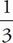# SAT Math Multiple Choice Question 100: Answer and Explanation

### Test Information

Question: 100

10. Melanie puts \$1,100 in an investment account that she expects will make 5% interest for each three-month period. However, after a year she realizes she was wrong about the interest rate and she has \$50 less than she expected. Assuming the interest rate the account earns is constant, which of the following equations expresses the total amount of money, x, she will have after t years using the actual rate?

• A. x = 1,100(1.04)4t
• B. x = 1,100(1.05)4t - 50
• C. x = 1,100(1.04)t/3
• D. x = 1,100(1.035)4t

A The formula for compound interest is A = P(1 + r)t, where P is the starting principle, r is the rate expressed as a decimal, and t is the number of times the interest is compounded. Melanie received less than 5% interest, so you can eliminate (B) because 1.05 = 1 + 0.05, indicating she was receiving 5% interest. You can also eliminate (C) because over the course of a year the interest is compounded 4 times, notof a time. Because Melanie invested \$1,100 at what she thought was 5% compounded 4 times (12 months in a year ÷ 3 months per period), she expected 1,100(1 + 0.05)4 = \$1,337.06 after a year. Instead, she has 1,337.06 - 50 = \$1,287.06 after one year. Because t is in years in the answer choices, make t = 1 in (A) and (D) and eliminate any choice which does not equal 1,287.06. Only (A) works.